# PSEB 4th Class Maths Solutions Chapter 2 Fundamental Operations on Numbers Revision Exercise

Punjab State Board PSEB 4th Class Maths Book Solutions Chapter 2 Fundamental Operations on Numbers Revision Exercise Questions and Answers.

## PSEB Solutions for Class 4 Maths Chapter 2 Fundamental Operations on Numbers Revision Exercise

1. Solve the following:

Question 1.Solution: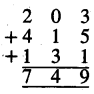Question 2.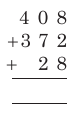Solution: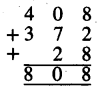Question 3.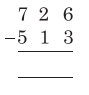Solution: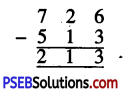Question 4.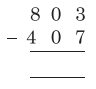Solution: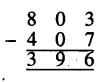2. Fill in the blanks:

Question 1.
15 + 26 = 26 +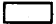Solution:
15

Question 2.
18 + 0 =Solution:
18Question 3.
13 × 1 =Solution:
13

Question 4.
25 × 0 =Solution:
0

Question 5.
32 – 0 =Solution:
32

Question 6.
9 ÷ 9 =Solution:
1

Question 7.
28 ÷ 4 =Solution:
7Question 8.
87 + 5 =Solution:
92

Question 9.
54 ÷ 9 =Solution:
6

Question 10.
16 ÷ 1 =Solution:
16

Question 11.
18 – 18 =Solution:
0

Question 12.
6 × 9 =Solution:
54Question 13.
0 ÷ 3 =Solution:
0

Question 14.
83 ÷ 83 =Solution:
1

3. Lets do:

Question 1.
In a school bus there are 32 boys and 16 girls. How many children are there in the bus?
Solution:
Number of boys = 32
Number of Girls = 16
Total no. of children in the bus = 48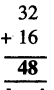Question 2.
Nirmal scored 93 marks in Punjabi and 98 marks in Maths. How much she secured in both the subjeds?
Solution:
Marks in Punjabi = 93
Marks in Maths = 98
Total marks secured in both the subjects = 191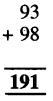4. Read the statements and solve:

Question 1.
Kamal bought 50 gas balloons. 19 balloons flew away. How many balloons were left behind?
Solution:
Total number of gas balloons = 50
Number of balloons flew away = – 19
Number of balloons left behind = 31Question 2.
Mankaran has 350 mangoes. He gave 145 mangoes to her sister Harkirat and rest are given to his friend Ramesh. How many mangoes did Ramesh get?
Solution:
Number of mangoes Mankaran has = 350
Number of mangoes he has given to Harkirat = – 145
Number of Mangoes got by Rairiesh = 205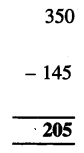5. Solve the following questions :

Question 1.
There are 58 toffees in a packet. How many toffees are in 16 such packets ?
Solution:
Number of toffees in a packet = 58
Number of toffees in 16 packets = 58 × 16
Hence, the total number of toffees in 16 packets =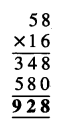Question 2.
There are seven days in a week. How many days are there in 52 week ?
Solution:
Number of days in a week = 7
Number of days in 52 weeks = 52 × 7
Hence, the total number of days in 52 weeks6. Understand and Solve the following:

Question 1.
5 men can sit in a car. What is the number of cars required for 20 men?
Solution:
5 men sits in the number of cars = 1
1 men sits in the number of cars = $$\frac{1}{5}$$
20 men sits in the number of cars
= $$\frac{1}{5}$$ × 20 = 4Question 2.
There are 368 cement bags in 8 trucks. Every truck has equal number of cement bags, find out cement bags in one truck.
Solution:
Number of cement bags in 8 trucks = 368
Number of cement bags in 1 truck = 368 ÷ 8 = 46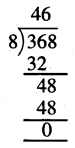∴ Number of cement bags in one truck = 46.

Multiple Choice Questions

Read the problems carefully and choose out the correct option from the given options.

Question 1.
There are 55 children in a park. 5 another children reach there. Now find out the total number of children in the park ? Recognise the basic operation :
(a) 55 – 5
(b) 55 + 5
(c) 55 ÷ 5
(d) 55 × 5.
Solution:
(b) 55 + 5.

Question 2.
If every child is given 5 toffees, then number of toffees required for 35 children. Recognise the basic operation :
(a) 35 – 5
(b) 35 + 5
(c) 35 ÷ 5
(d) 35 × 5.
Solution:
(d) 35 × 5Question 3.
The sum of two numbers is 120, if one number is 40, find he other number. Recognise the basic operation :
(a) 120 – 40
(b) 120 × 40
(c) 120 ÷ 40
(d) 120 + 40.
Solution:
(a) 120 – 40.

Question 4.
In 8 boxes 264 books are arranged equally. What are the number of books in each box. Recognise the basic operation :
(a) 264 – 8
(b) 264 ÷ 8
(c) 264 × 8
(d) 264 + 8.
Solution:
(b) 264 ÷ 8.

Question 5.
There are 500 bricks in a block. If we sell 200 bricks how many bricks were left behind ? Recognise the basic operation :
(a) 500 – 200
(b) 500 × 200
(c) 500 ÷ 200
(d) 500 + 200.
Solution:
(a) 500 – 200.Question 6.
Ten people planted 480 plants. Each one of them planted same number of plants. Find out the number of plants planted by one person. Recognize the basic operation.
(a) 480 – 10
(b) 480 ÷ 10
(c) 480 × 10
(d) 480 + 10.
Solution:
(b) 480 ÷ 10